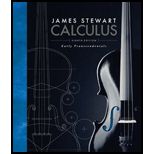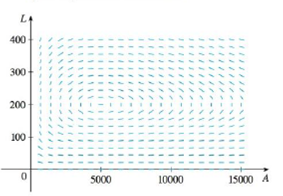# Populations of aphids and ladybugs are modeled by the equations d A d t = 2 A − 0.01 A L d L d t = − 0.5 L + 0.0001 A L (a) Find the equilibrium solutions and explain their significance. (b) Find an expression for dL / dA. (c) The direction field for the differential equation in part (b) is shown. Use it to sketch a phase portrait. What do the phase trajectories have in common? (d) Suppose that at time t = 0 there are 1000 aphids and 200 ladybugs. Draw the corresponding phase trajectory and use it to describe how both populations change. (e) Use part (d) to make rough sketches of the aphid and ladybug populations as functions of t . How are the graphs related to each other?### Calculus: Early Transcendentals

8th Edition
James Stewart
Publisher: Cengage Learning
ISBN: 9781285741550

#### Solutions

Chapter
Section### Calculus: Early Transcendentals

8th Edition
James Stewart
Publisher: Cengage Learning
ISBN: 9781285741550
Chapter 9.6, Problem 10E
Textbook Problem
8 views

## Populations of aphids and ladybugs are modeled by the equations d A d t = 2 A − 0.01 A L d L d t = − 0.5 L + 0.0001 A L (a) Find the equilibrium solutions and explain their significance.(b) Find an expression for dL/dA.(c) The direction field for the differential equation in part (b) is shown. Use it to sketch a phase portrait. What do the phase trajectories have in common?(d) Suppose that at time t = 0 there are 1000 aphids and 200 ladybugs. Draw the corresponding phase trajectory and use it to describe how both populations change.(e) Use part (d) to make rough sketches of the aphid and ladybug populations as functions of t. How are the graphs related to each other?

(a)

To determine

To find: The equilibrium solution and their significance.

### Explanation of Solution

Given:

The equations are dAdt=2A0.01AL and dLdt=0.5L0.0001AL .

Calculation:

Consider A and L be constants.

Differentiate with respect to t,

From given,

0=2A0.01AL

0=0.5L0.0001AL

Solving further,

0=A(20

(b)

To determine

To find: An expression for dLdA .

(c)

To determine

To draw: The phase trajectories.

(d)

To determine

To draw: The phase trajectories.

(e)

To determine

To draw: The rough sketch for ladubugs and aphids.

### Still sussing out bartleby?

Check out a sample textbook solution.

See a sample solution

#### The Solution to Your Study Problems

Bartleby provides explanations to thousands of textbook problems written by our experts, many with advanced degrees!

Get Started

Find more solutions based on key concepts
Find the limit. limx1sin(x1)x2+x2

Single Variable Calculus: Early Transcendentals, Volume I

In Exercises 107-120, factor each expression completely. 113. 3x2 6x 24

Applied Calculus for the Managerial, Life, and Social Sciences: A Brief Approach

Factor: 7xy21y

Elementary Technical Mathematics

Using for |x| < 1,

Study Guide for Stewart's Single Variable Calculus: Early Transcendentals, 8th

Rewritten in reverse order,

Study Guide for Stewart's Multivariable Calculus, 8th

Reminder Round all answers to two decimal places unless otherwise indicated. The Doyle Log Rule is a formula th...

Functions and Change: A Modeling Approach to College Algebra (MindTap Course List)

49. The admissions officer for Clearwater College developed the following estimated regression equation relatin...

Modern Business Statistics with Microsoft Office Excel (with XLSTAT Education Edition Printed Access Card) (MindTap Course List)

In Problems 4150 use the Laplace transform to solve the given integral equation or integrodifferential equation...

A First Course in Differential Equations with Modeling Applications (MindTap Course List)